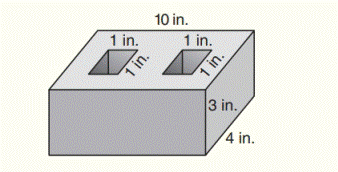Chapter 9.CR, Problem 29CRElementary Geometry For College St...

7th Edition
Alexander + 2 others
ISBN: 9781337614085

Solutions

Chapter
SectionElementary Geometry For College St...

7th Edition
Alexander + 2 others
ISBN: 9781337614085
Textbook Problem

Find the volume of cement used in the block shows.To determine

To find:

The volume of cement used in the block.

Explanation

Formula used:

Volume of rectangular prism:

V=lwh

Given:

Find the volume of cement used in the block shows.

Calculation:

The cement used in the block is containing a large rectangular prism and two smaller rectangular prisms.

The length of large rectangular prism is l1=10 in.

The width of large rectangular prism is w1=4 in.

The height of large rectangular prism is h1=3 in.

Also,

The length of small rectangular prism is l2=1 in.

The width of small rectangular prism is w2=1 in.

In the figure, the height of the large rectangular prism is equal to the height of the small rectangular prism

The height of small rectangular prism is h2=3 in.

The volume of cement used in the block is,

Still sussing out bartleby?

Check out a sample textbook solution.

See a sample solution

The Solution to Your Study Problems

Bartleby provides explanations to thousands of textbook problems written by our experts, many with advanced degrees!

Get Started

Simplify: 728

Elementary Technical Mathematics

{x+y+2z=62x+5z=12x+2y+3z=9

Precalculus: Mathematics for Calculus (Standalone Book)

Rationalize the denominator of xx42 and simplify.

Mathematical Applications for the Management, Life, and Social Sciences

Use the guidelines of this section to sketch the curve. y = x5/3 5x2/3

Single Variable Calculus: Early Transcendentals, Volume I

9. List four mortgage loan closing costs. (14-4)

Contemporary Mathematics for Business & Consumers